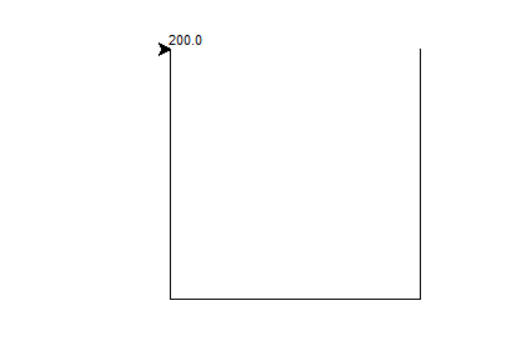• 类似的需求应该比较多，这里的核心点是关于两个坐标点之间距离计算，之前没用过这个东西，刚好今天有个这样的需求，记录下。 import requests import psycopg2 import pandas as pd from math import radians, ...
背景：有几个门店和大厦，看看这几个门店和大厦旁边有没有快递柜
数据：门店和大厦只有地址数据，快递柜系统有地址数据和经纬度数据
类似的需求应该比较多，这里的核心点是关于两个坐标点之间距离的计算，之前没用过这个东西，刚好今天有个这样的需求，记录下。
import requests
import psycopg2
import pandas as pd
from math import radians, cos, sin, asin, sqrt

#返回地址的坐标
#使用高德地图的api,获取地址的经纬度，注意key用自己的key,这里的key是瞎写的
base = 'http://restapi.amap.com/v3/geocode/geo'
response = requests.get(base, parameters)
return lon,lat

#返回系统所有的深圳快递柜的坐标
def get_cabinet_coordinate():
conn = psycopg2.connect(database="database",
user="root",
host="localhost",
port="443")
cursor = conn.cursor()
sql = "select  * from  t_station where  city_code = 440300"
cursor.execute(sql)
df = pd.DataFrame(cursor.fetchall())
cursor.close()
df = df.iloc[:,[11,3,4,5,6]]
return df

#获取两个坐标点的距离
def get_distance(lon1, lat1, lon2, lat2):
# 将十进制度数转化为弧度
lon1, lat1, lon2, lat2 = map(radians, [lon1, lat1, lon2, lat2])

# haversine公式
dlon = lon2 - lon1
dlat = lat2 - lat1
a = sin(dlat/2)**2 + cos(lat1) * cos(lat2) * sin(dlon/2)**2
c = 2 * asin(sqrt(a))
r = 6371 # 地球平均半径，单位为公里
#print(c * r * 1000)
return c * r * 1000

if __name__ == '__main__':
df = get_cabinet_coordinate()
lst = list()
#print(lon1,lat1)
for i in df.values:
lon2 = i
lat2 = i
distance = get_distance(lon1, lat1, lon2, lat2)
if distance<=3000:
lst.append({
'lon1':lon1,             #经度
'lat1':lat1,             #维度
'sn':i,               #快递柜sn编码
'lon2':i,             #经度
'lat2':i,             #维度
'distance':distance      #快递柜和分析地址距离
})
#print(dic)
df = pd.DataFrame(lst)
df.to_excel(r'C:\Users\James\Desktop\地域数据_距离.xlsx')
print('数据保存成功')
print(df.head())

展开全文• 话不多说，直接上代码 def haversine(lng1, lat1, lng2, lat2):...# 经度1，纬度1，经度2，纬度2 （十进制度数）计算两经纬度坐标点的距离 文章摘自 https://blog.csdn.net/vernice/article/details/46581361
话不多说，直接上代码
def haversine(lng1, lat1, lng2, lat2):
lng1, lat1, lng2, lat2 = map(radians, [lng1, lat1, lng2, lat2])
dlon = lng2 - lng1
dlat = lat2 - lat1
a = sin(dlat/2)**2 + cos(lat1) * cos(lat2) * sin(dlon/2)**2
c = 2 * asin(sqrt(a))
r = 6371 # 地球平均半径，单位为公里
return c * r * 1000
# 经度1，纬度1，经度2，纬度2 （十进制度数）计算两经纬度坐标点的距离
文章摘自https://blog.csdn.net/vernice/article/details/46581361
展开全文• 今天小编就为大家分享一篇python计算两个地址之间的距离方法，具有很好的参考价值，希望对大家有所帮助。一起跟随小编过来看看吧
• import math ipt = input('请输入x1,y1,x2,y2，以逗号隔开:') data = ipt.split(',') data = [int(x) for x in ...print(data, ',', data, '与', data, ',', data, '之间的距离为：', result)
import math

ipt = input('请输入x1,y1,x2,y2，以逗号隔开:')
data = ipt.split(',')
data = [int(x) for x in data]
result = math.sqrt(
math.pow(
data -
data,
2) +
math.pow(
data -
data,
2))
print(data, ',', data, '与', data, ',', data, '之间的距离为：', result)

展开全文• 4，write()方法写入计算距离 示例代码如下，IDLE环境下可直接运行 import turtle import math x1,y1 = 100,100 x2,y2 = 100,-100 x3,y3 = -100,-100 x4,y4 = -100,100 turtle.penup() turt...
1，导入turtle模块 2，导入math模块 3，调用math.sqrt()开平方的方法 4，write()方法写入计算的距离 示例代码如下，IDLE环境下可直接运行
import turtle
import math

x1,y1 = 100,100
x2,y2 = 100,-100
x3,y3 = -100,-100
x4,y4 = -100,100

turtle.penup()
turtle.goto(x1,y1)
turtle.pendown()
turtle.goto(x2,y2)
turtle.goto(x3,y3)
turtle.goto(x4,y4)

distance = math.sqrt((x1-x4)**2 + (y1-y4)**2)
turtle.write(distance)


运行结果如下：以上
如有错误，欢迎指正
欢迎粘贴复制 评论转发点赞
轻轻一点 好运连连
展开全文海龟绘图 折线图
• * 计算两个坐标之间的距离(米) * @param float $fP1Lat 起点(纬度) * @param float$fP1Lon 起点(经度) * @param float $fP2Lat 终点(纬度) * @param float$fP2Lon 终点(经度) * @return int */ function ...
• 点的坐标是(x1, y1)和(x2, y2) 则点之间的距离公式为 d=√[(x1-x2)²+(y1-y2)²] 注意特例： 当x1=x2时 点间距离为|y1-y2| 当y1=y2时 点间距离为|x1-x2| 中点坐标：midpoint(X,Y) X=(X1+X2)/2 Y...
• 夹角示意图说明： ...# 两个经纬度之间的距离 def LatLng2Dist(LatZero,LngZero,Lat,Lng): ra = 6378140 # radius of equator: meter rb = 6356755 # radius of polar: meter flatten = (ra - rb) / ra # Parti
• # -*- coding: utf-8 -*- # -------------------------------------- # DATE: 2018-12-09 # OUT: calculate_line_distance_amap.py # DEV: yulong # -------------------------------------- import sys ...高德地图
• 一、两个坐标之间距离计算 参考链接： python实现 1、Python 根据地址获取经纬度及求距离 2、python利用地图两个点的经纬度计算两点间距离 　LBS 球面距离公式 美团app筛选“离我最近” 之 地理空间距离计算...
• ## 用Python如何计算两点间距离

万次阅读 多人点赞 2020-03-02 17:27:36
Python如何计算两点间距离？ 第一步，我们先来看一下两点间的距离公式。 两点间距离公式： ？？？这是啥？ 是不是方法一还能看懂，方法二却一脸懵逼？ 请仔细看注释， 也就是说，根号2还可以写成2的...
• #对于坐标为同一点时,返回点与点的距离 if line_point1 == line_point2: point_array = np.array(point ) point1_array = np.array(line_point1) return np.linalg.norm(point_array -point1_array ) #...python
• 这种文章本来网上一搜一大把，但有的计算结果是错的，遂找到个计算正确的，记录一下。 参考原文：LBS 球面距离公式 from math import sin, asin, cos, radians, fabs, sqrt EARTH_RADIUS=6371 # 地球平均半径，...lbs 地图
• 知道两点坐标，怎么计算两点方向的方位角？ 答：首先计算坐标增量dx，dy(两个对应坐标分量相减，终点的减始点的)。 若dx,dy中有一个为零时，根据另一个的正负决定方位角（0，90，180，270这四个中的一个，可画坐标...
• 这是来自知乎上的问题。问我的时候，恰好我在写一和向量计算相关的文章，于是灵光乍现，顺手写了这样一答案。该算法未经严格验证，请谨慎参考。numpy
• 碰到一道练习题：求解距离矩阵，首先生成一百个二维坐标点，计算任意两个坐标点的距离。我一开始看到这题目是一脸懵逼，然后上网找了一下，更是二脸懵逼。生成一百个二维坐标点，我首先就是想去生成一个1010的二维...numpy
• /** * 计算两组经纬度坐标 之间的距离 * params ：lat1 纬度1； lng1 经度1； lat2 纬度2； lng2 经度2； len_type （1:m or 2:km); * return m or km */ /** * 根据经纬度查询距离 * @param lng1 经度 * @param lat...
• 计算两个GPS经纬度之间的距离Python 实现） 用到的库 from geographiclib.geodesic import Geodesic 代码实现 from geographiclib.geodesic import Geodesic coords_1 = (float(lat1_in_degrees), float(long1_in_...
• #定义一个二维平面上的点，包含x轴y轴的坐标以及计算两个点之间距离的方法。 #不一定必须写 import math #定义一个类，用于计算两点之间距离、提供修改坐标函数、提供输出结果函数 class Distance: def __init__...
• 已知有N个模块，每个模块里包含四个x和y坐标。 将每个模块中的每个坐标计算与其他模块中每个坐标的欧式距离。 找到该坐标距离最近的其他模块的坐标，输出配对结果。 使用python该如何进行？
• 1. 欧氏距离(Euclidean Distance) ...计算公式是两个矩阵中对应元素之差的平方和再开方。 python实现： import numpy as np x = np.array([[1, 2, 3, 4],[5, 6, 7, 8]]) y = np.array([[5, 6, 7, 8],[1, 2, ...
• 代码如下： ...给定两个地址的坐标计算返回两地相距多少公里 demo: address1=成都 lon1 = 104.071000 lat1 = 30.670000 address2=宜宾 104.622000 lat2 = 28.765000 ''' def get_address_dis...
• 最近项目中需要计算个坐标点与多个点的距离，发现用Python来实现效率很低。经同事推荐，将这些坐标点存入了Mysql数据库，然后用数据库自带的方法st_distance_sphere计算距离。经过比较发现确实效率提高了很多，...SQL
• 初始化10个坐标计算个坐标与其他坐标的欧式距离 import numpy as np x = np.random.rand(10,2) #计算10坐标之间的差值（欧氏距离） L = np.sum((x[:,np.newaxis,:] - x[np.newaxis,:,:]) ** 2, axis = 1) "...最近邻
• 近期在用 OpenCV 时需要... 点到直线距离由于项目中得到点的坐标最容易，因此采用向量法进行所有的数学计算最清晰明了。点到直线距离就采用向量法推导。 推导过程： 代码实现：array_longi = np.array([x2-x1, y...
• 时隔几月，我又来了。 只是我还不太懂的问题，我想先记录一下，等回头再重新思考。 直接放题目和答案了。 另外放一位作者的讲解 https://blog.csdn.net/qq_44788797/article/details/105464405numpy anaconda
• 处理地图数据时，经常需要用到两个地理位置间的距离。比如A点经纬度（110.0123, 23.32435），B点经纬度（129.1344,25.5465），求AB两点之间的距离。我们可以用haversine()函数求出距离结果。Python版本的haversine()...haversine 度数转弧度
• 目前各个地图的经纬度坐标还是有差异的，为了开发人员能方便的进行坐标间的转化，顺带分享一经纬度坐标转换的api， 点击打开链接 还是很不错的，但是好像一天有几千次的限制，不太记得了，管他呢，能用就行。 ...api...

# python计算两个坐标的距离python 订阅